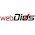# Python program to find factorial without recursion

• Python program to find factorial of a number without using recursion in python.
• Algorithm to find factorial of a number without using recursion function using python.

#1: Python program to find factorial of a given number without using recursion
1. #Factorial without recursion

2. n=int(input("Enter the number: "))
3. fact=1
4. if n<0:
5.     print("factorial doesn't exist for negative numbers")
6. else:
7.     for i in range(1,n+1):
8.         fact=fact*i
9.     print("factorial of", n, "is", fact)

Output:

#### Instance Of Java

1.